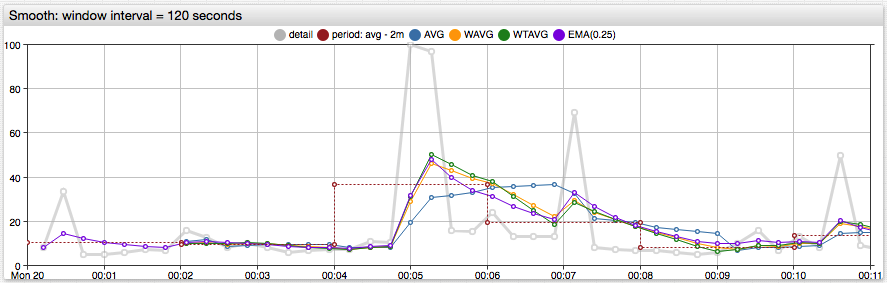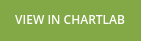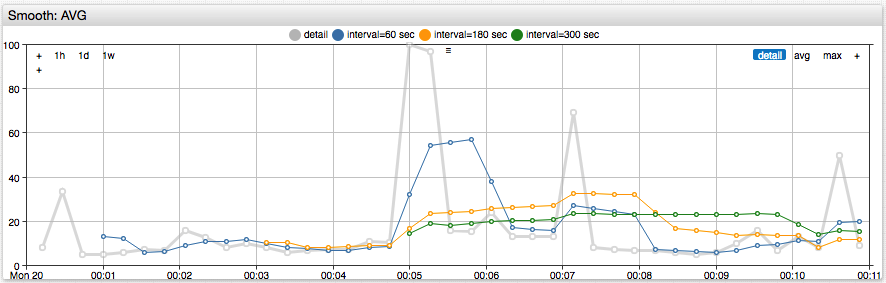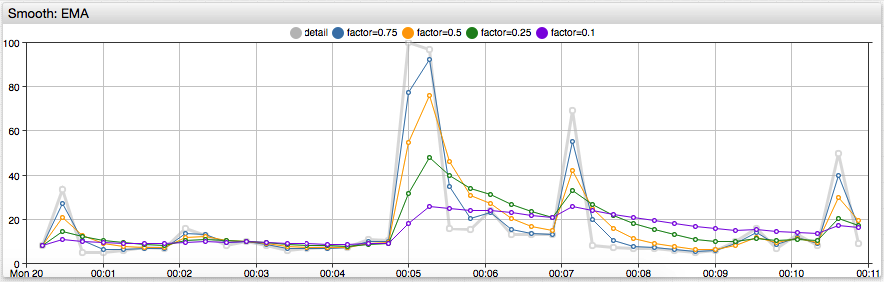# # Smoothing Time Series## # Overview

Smoothing is a transformation that reduces the noise in an underlying time series. Unlike period aggregation which returns series with new timestamps set to period start times, the smoothing transformation retains the original timestamps.

For each timestamp in the underlying series, the smoothed value is calculated in two steps:

• A sequence of preceding samples up to the current timestamp is retrieved from the underlying series. This sequence ends with the current sample and is called a rolling (or sliding) window.
• A new value at the current timestamp is calculated by applying a smoothing function, such as average, to the retrieved sequence.

Supported smoothing functions:

• `AVG`: Average
• `COUNT`: Count
• `SUM`: Sum
• `WAVG`: Weighted Average
• `WTAVG`: Weighted-time Average
• `EMA`: Exponential Moving Average

The size of the rolling window is based on either time duration or the number of samples. Window size is controlled by the `interval` and `count` settings.

The example below calculates the simple moving average with a 15 minute rolling window.

``````"smooth": {
"type": "AVG",
"interval": {"count": 15, "unit": "MINUTE"}
}
``````

The implemented smoothing functions differ in how they assign weight to samples in the sliding window. Simple average `AVG` assigns equal weight to all samples. Weighted average functions take the sample index or time distance between samples into account and assign greater weight to more recent samples.

## # Fields

Name Type Description
`type` string [Required] Smoothing function.
Available functions: `AVG`, `COUNT`, `SUM`, `WAVG`, `WTAVG`, `EMA`.
`count` number Number of samples in a count-based rolling window.
`interval` object Duration of a time-based window specified with `count` and time `unit`.
For example: `{"count": 3, "unit": "HOUR"}`.
Supported units: `MILLISECOND`, `SECOND`, `MINUTE`, `HOUR`.
`minimumCount` number Minimum number of samples in a window required to apply the smoothing function.
Default value is `1`.
If a window is incomplete (count is below minimum), the smoothing function returns `incompleteValue` for the current timestamp.
`incompleteValue` string Number returned in an incomplete window for the current timestamp if the sample count is below `minimumCount`.
Possible values: `null` (default), `NaN`, or a constant numeric value.
`null` values are omitted from the response.

Parameters `count` and `interval` are ignored by the `EMA` function which weighs all loaded samples.

The longer the time window, the more smoothing is performed on the returned series.## # Smoothing Process

Received series samples are processed sequentially in ascending time order. The ordered sequence is called the rolling window or a sliding window. Initially the window is empty.

For each series sample the following steps are executed sequentially:

1. If the sample value is `NaN`, include the sample in the response unchanged. Skip remaining steps.
2. Append the sample to the end of the window.
3. Remove outdated samples:
• For time-based windows: Remove any samples with timestamp equal to or earlier than the current timestamp minus the interval.
• For count-based windows: Remove the first (oldest) sample if window length exceeds `count`.
4. If the number of samples is below `minimumCount`, return the value specified in the `incompleteValue` parameter.
5. Return the value calculated by the smoothing function.

The timestamp of the returned samples is the same as the timestamp of the input sample.

## # Smoothing Functions

### # Average

`type = AVG`

Calculates average value within rolling window. Sum of values divided by number of values.

Window values:Calculation formula:### # Count

`type = COUNT`

Calculates the count of values in the rolling window.

### # Sum

`type = SUM`

Calculates the sum of values in the rolling window.

### # Weighted Average

`type = WAVG`

Calculates weighted average where the weight of the value equals the sample index.

Window values:Calculation formula:### # Weighted Time Average

`type = WTAVG`.

Calculates weighted average where the weight of the sample value is equal to the distance between the first timestamp and the current sample timestamp.

Window samples, with timestamps measured in milliseconds:Calculation formula:The function returns sample value () if.

### # Exponential Moving Average

`type = EMA`

Calculates exponentially smoothed weighted average. The function uses a window which consists of all samples preceding the current timestamp, therefore it does not require `count` and `interval` parameters. The contribution of a sample to the calculated smoothed value decreases exponentially for more distant samples.

#### #`EMA` Specific Fields

Name Type Description
`factor` number Smoothing factor. A number within the `(0, 1)` range.
A smaller smoothing factor decreases variance.
Default value: `0.25`
`range` number Controls steepness of the smoothing function.
Typically used to smooth irregular series.
A larger range decreases variance.Input series samples:The smoothed series contains samples with the same timestamps:• Calculations using `factor`whereis the value of smoothing `factor`.

• Calculations using `range`

The calculation applies the same formulas `(1)` and `(2)`, using a `factor` calculated based on series timestamps and the specified `range`:whereis the value of `range`.

For regular series, `range` can be calculated from `factor`:whereis the time interval between consecutive samples, measured in milliseconds.

If the interval between samples is `1000` milliseconds, and smoothing factor is `0.5`, the `range` is `1443`.

• A. Eckner, Algorithms for Unevenly Spaced Time Series: Moving Averages and Other Rolling Operators, Section 4.1, `EMA_next`.
• U. Muller, Specially Weighted Moving Averages with Repeated Application of the EMA Operator, Formulas `2.7`-`2.14`.